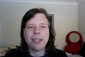# Electrical basic measurements and valuesElectricity has three measurements, the volt, the amp, and the watt.

1 watt = 1 volt * 1 amp

Loosely speaking, the volts (voltage) of an electrical current measures the strength of that current. Similarly speaking loosely, the amps (amperage) of that electrical current measures the quantity of electrons flowing by. Household electricity (in the U.S.) runs at 120 volts, yes? In an electric vehicle, roughly speaking, the voltage the system runs at directly corresponds with the "speed" of that vehicle, while the amps available directly corresponds with the range. There are exceptions but that is a good rough correspondence.

The next electrical measurement of interest is "kilowatt-hours".

1 kilowatt-hour = 1 kilowatt used over 1 hour
1 kilowatt = 1,000 watts

To bring this to every day terms, the typical lightbulb is 100 watts, yes? Well, unless you're like me and use compact flourescent lighting, in which case the lightbulb in question uses about 40 watts. In any case, if you have ten 100 watt lightbulbs, that is 1,000 watts. Leave them running for an hour, and that is a kilowatt-hour. By modern electricity rates it will cost you in the neighborhood of ten cents for that electricity. As an electric vehicle moves down the road, it consumes electricity. Say the vehicle has a 120 volt electrical system, and uses 30 amps to cruise, therefore the vehicle cruises at 3.6 kilowatts. If the vehicle is run for an hour, it consumes 3.6 kilowatt hours of electricity.

Batteries are rated in voltage and amp-hour storage capacity. Obviously this is the kilowatt-hours of storage capacity. By the way, these measures also relate to batteries in cell phones and laptop computers, and directly relate to the "talk time" capacity of either one. In actuality the true capacity of a battery pack relates to the usage model.

When a battery company gives an "amp-hour" rating for a battery, they also specify the rate of discharge. Often the rating is given for a "20-hour" discharge, meaning that the rate amps are drawn from the battery is geared towards discharging the battery in a 20-hour period. When batteries are used at a higher rate the actual amp-hours that can be drawn from the battery often is lower than its 20-hour discharge rate implies.

<< Need a chart here showing the typical pattern - also explain C/20 and C/1 nomenclature >>

## Who's online

There are currently 0 users online.

• momo4gou
• wildfire_one
• Jasmine
• Jordyn
• JCunnius